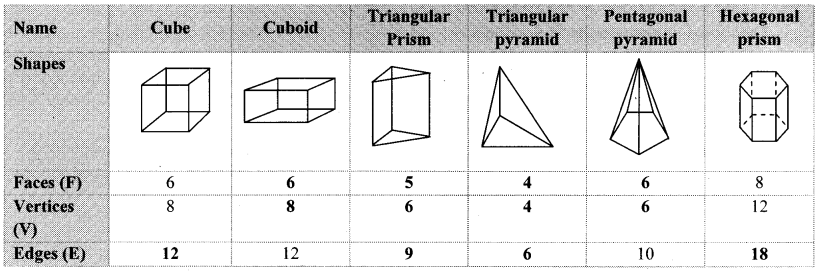# Maharashtra Board 8th Class Maths Practice Set 16.3 Solutions Chapter 16 Surface Area and Volume

Balbharti Maharashtra State Board Class 8 Maths Solutions covers the Practice Set 16.3 8th Std Maths Answers Solutions Chapter 16 Surface Area and Volume.

## Practice Set 16.3 8th Std Maths Answers Chapter 16 Surface Area and Volume

Question 1.
Find the volume of the cylinder if height (h) and radius of the base (r) are as given below.
i. r = 10.5 cm, h = 8 cm
ii. r = 2.5 m, h = 7 m
iii. r = 4.2 cm, h = 5 cm
iv. r = 5.6 cm, h = 5 cm
Solution:
i. Given: r = 10.5 cm and h = 8 cm
To find: Volume of the cylinder
Volume of the cylinder = πr²h
= $$\frac { 22 }{ 7 }$$ x 10.5 x 10.5 x 8
= 22 x 1.5 x 10.5 x 8
= 2772 cc
∴ The volume of the cylinder is 2772 cc.

ii. Given: r = 2.5 m and h = 7 m
To find: Volume of the cylinder
Volume of the cylinder = πr²h
= $$\frac { 22 }{ 7 }$$ x 2.5 x 2.5 x 7
= 22 x 2.5 x 2.5
= 137.5 cu.m
∴ The volume of the cylinder is 137.5 cu.m.

iii. Given: r = 4.2 cm and h = 5 cm
To find: Volume of the cylinder
Volume of the cylinder = πr²h
= $$\frac { 22 }{ 7 }$$ x 4.2 x 4.2 x 5
= 22 x 0.6 x 4.2 x 5
= 277.2 cc
∴ The volume of the cylinder is 277.2 cc.

iv. Given: r = 5.6 cm and h = 5 cm
To find: Volume of the cylinder
Volume of the cylinder = πr²h
= $$\frac { 22 }{ 7 }$$ x 5.6 x 5.6 X 5 7
= 22 x 0.8 x 5.6 x 5
= 492.8 cc
∴ The volume of the cylinder is 492.8 cc.

Question 2.
How much iron is needed to make a rod of length 90 cm and diameter 1.4 cm?
Solution:
Given: For cylindrical rod: length of rod (h) = 90 cm, and
diameter (d) = 1.4 cm
To find: Iron required to make a rod
diameter (d) = 1.4 cm
∴ radius (r) = $$\frac{\mathrm{d}}{2}=\frac{1.4}{2}$$ = 0.7 cm
Volume of rod = πr²h
= $$\frac { 22 }{ 7 }$$ x 0.7 x 0.7 x 90
= 22 x 0.1 x 0.7 x 90
= 138.60 cc
∴ 138.60 cc of iron is required to make the rod.

Question 3.
How much water will a tank hold if the interior diameter of the tank is 1.6 m and its depth is 0.7 m?
Solution:
Given: interior diameter of the tank (d) = 1.6 m
and depth (h) = 0.7 m
To find: Capacity of the tank
interior diameter of the tank (d) = 1.6 m
∴ Interior radius (r) = $$\frac{\mathrm{d}}{2}=\frac{1.6}{2}$$
= 0.8 m
= 0.8 x 100
…[∵ 1m = 100cm]
= 80cm
h = 0.7 m = 0.7 x 100 = 70 cm
Capacity of the tank = Volume of the tank = πr²h
= $$\frac { 22 }{ 7 }$$ x 80 x 80 x 70
= 22 x 80 x 80 x 10
= 1408000 cc
= $$\frac { 1408000 }{ 1000 }$$
…[∵1 litre = 1000 cc]
= 1408 litre
∴The tank can hold 1408 litre of water.

Question 4.
Find the volume of the cylinder if the circumference of the base of cylinder is 132 cm and height is 25 cm.
Solution:
Given: Circumference of the base of cylinder = 132 cm and height (h) = 25 cm
To find: Volume of the cylinder
i. Circumference of base of cylinder = 2πr
∴132 = 2 x $$\frac { 22 }{ 7 }$$ x r
∴$$\frac{132 \times 7}{2 \times 22}=r$$
∴$$\frac{6 \times 7}{2}=r$$
∴3 x 7 = r
∴r = 21 cm

ii. Volume of the cylinder = πr²h
= $$\frac { 22 }{ 7 }$$ x 21 x 21 x 25
= 22 x 3 x 21 x 25
= 34650 cc
∴ The volume of the cylinder is 34650 cc.

Maharashtra Board Class 8 Maths Chapter 16 Surface Area and Volume Practice Set 16.3 Intext Questions and Activities

Question 1.
Leonard Euler, discovered an interesting formula regarding the faces, vertices and edges of solid figures.
Count and write the faces, vertices and edges of the following figures and complete the table. From the table verify Euler’s formula, F + V = E + 2. (Textbook pg. No. 113)Solution:From the above table, F + V = E + 2 i.e. Euler’s formula is verified.